## 1. CrossEntropy Loss

CrossEntropyLoss：

$$L = -\sum_{c=1}^{M} y_c log(p_c)$$

Pytorch - 官方损失函数汇总 - 3/4/6

Pytorch 示例：

import torch
import torch.nn as nn
import torch.nn.functional as F

#二值交叉熵，这里输入要经过sigmoid处理
nn.BCELoss(F.sigmoid(input), target)

#多分类交叉熵, 用这个 loss 前面不需要加 Softmax 层
nn.CrossEntropyLoss(input, target)

## 2. Weights CrossEntropy Loss

Weights CrossEntropyLoss：

$$L = -\sum_{c=1}^{M} w_c y_c log(p_c)$$

## 3. Focal Loss

Focal Loss 是在 RetinaNet 论文中提出来解决难易样本数量不均衡问题的.

Focal Loss 论文理解及公式推导 - AIUAI

One-Stage的目标检测器通常会产生 10k 数量级的框，但只有极少数是正样本，正负样本数量非常不平衡. 在计算分类的时候常用的交叉熵损失函数的公式如下：

$$CE(x, y) = \begin{cases} -log(p) &\text{if } y=1 \\ -log(1-p) &\text{otherwise } \end{cases}$$

$$CE = \begin{cases} -\alpha log(p) &\text{if } y=1 \\ -(1-\alpha)log(1-p) &\text{if } y=0 \end{cases}$$

RetinaNet 论文认为 易分样本(即，置信度高的样本)对模型的提升效果非常小，模型应该主要关注与那些难分样本 . 所以提出只需将高置信度样本的损失降低一些即可. 即 Focal Loss：

$$FL = \begin{cases} -(1-p)^{\gamma} log(p) &\text{if } y=1 \\ -p^{\gamma}log(1-p) &\text{if } y=0 \end{cases}$$

$$FL = \begin{cases} -\alpha(1-p)^{\gamma} log(p) &\text{if } y=1 \\ -(1-\alpha)p^{\gamma}log(1-p) &\text{if } y=0 \end{cases}$$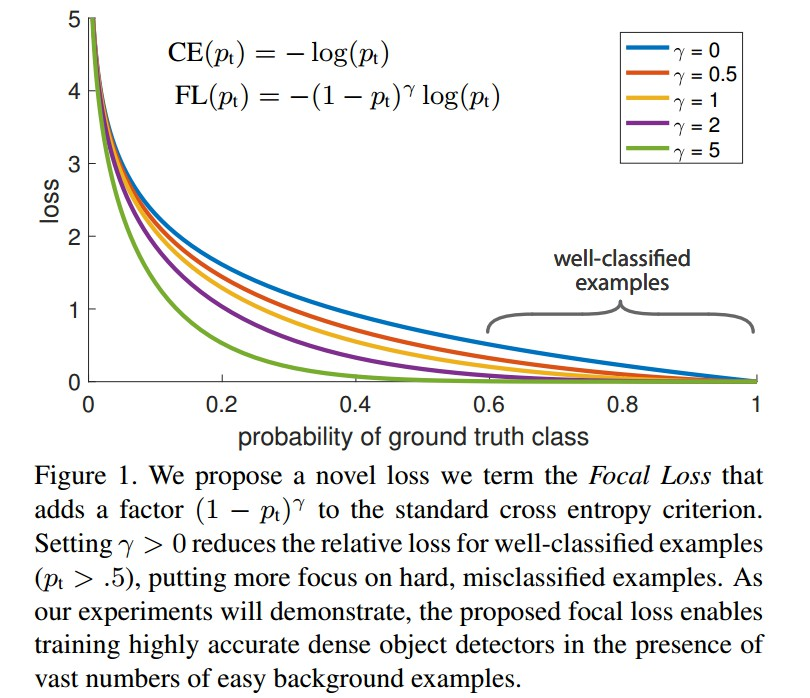Focal Loss的Pytorch代码实现如下：

class FocalLoss(nn.Module):
def __init__(self, gamma=0, alpha=None, size_average=True):
super(FocalLoss, self).__init__()
self.gamma = gamma
self.alpha = alpha
if isinstance(alpha,(float,int,long)):
self.alpha = torch.Tensor([alpha,1-alpha])
if isinstance(alpha,list):
self.alpha = torch.Tensor(alpha)
self.size_average = size_average

def forward(self, input, target):
if input.dim()>2:
# N,C,H,W => N,C,H*W
input = input.view(input.size(0),input.size(1),-1)
# N,C,H*W => N,H*W,C
input = input.transpose(1,2)
# N,H*W,C => N*H*W,C
input = input.contiguous().view(-1,input.size(2))
target = target.view(-1,1)

logpt = F.log_softmax(input)
logpt = logpt.gather(1,target)
logpt = logpt.view(-1)
pt = Variable(logpt.data.exp())

if self.alpha is not None:
if self.alpha.type()!=input.data.type():
self.alpha = self.alpha.type_as(input.data)
at = self.alpha.gather(0,target.data.view(-1))
logpt = logpt * Variable(at)

loss = -1 * (1-pt)**self.gamma * logpt
if self.size_average:
return loss.mean()
else:
return loss.sum()

## 4. Dice Loss

 - Dice系数

Dice系数是用来度量集合相似度的度量函数，通常用于计算两个样本的相似度(值范围为 [0, 1])：

$$s = \frac{2|X \bigcap Y|}{|X \bigcup Y|}$$

$$s = \frac{2TP}{2TP+FN+FP}$$

 - DiceLoss

$$Loss = 1 - \frac{2|X \bigcap Y|}{|X \bigcup Y|}$$

Dice Loss使用与样本极度不均衡的情况，如果一般情况下使用Dice Loss会回反向传播有不利的影响，使得训练不稳定.

 - 训练分割网络，例如FCN，UNet是选择交叉熵Loss还是选择Dice Loss?

(1) - 假设用 $p$ 表示预测值，$t$ 表示真实标签值，那么交叉熵损失函数关于的梯度形式类似于 $p-t$；而Dice Loss的值是 $1 - \frac{2pt}{p^2 + t^2}$ 或 $1 - \frac{2pt}{p + t}$，其关于 $p$ 的梯度形式为 $\frac{2t^2}{(p+t)^2}$ 或 $\frac{2t(t^2-p^2)}{(p^2+t^2)^2}$. 可以看到在极端情况下即 $p$ 和 $t$ 都很小的时候，计算得到的梯度值可能会非常大，即会导致训练十分不稳定.

(2) - 另外一种解决类别不平衡的方法是简单的对每一个类别根据赋予不同的权重因子(如对数量少的类别赋予较大的权重因子)，使得样本数量不均衡问题得到缓解(上面已经介绍过了，就是带权重的交叉熵Loss).

DiceLoss 的实现：

import torch.nn as nn
import torch.nn.functional as F

class SoftDiceLoss(nn.Module):
def __init__(self, weight=None, size_average=True):
super(SoftDiceLoss, self).__init__()

def forward(self, logits, targets):
num = targets.size(0)
#为了防止除0的发生
smooth = 1

probs = F.sigmoid(logits)
m1 = probs.view(num, -1)
m2 = targets.view(num, -1)
intersection = (m1 * m2)

score = 2. * (intersection.sum(1) + smooth) / (m1.sum(1) + m2.sum(1) + smooth)
score = 1 - score.sum() / num
return score

## 5. IoU Loss

IoU Loss 和 Dice Loss 一样，属于 metric learning 的衡量方式，其定义：

$$L = 1 - \frac{A \bigcap B}{A \bigcup B}$$

IoU Loss 和 Dice Loss 一样存在训练过程中不稳定的问题.

IoU Loss 在图像分割任应用较少. IoU Loss 的代码只需在 Dice Loss 的基础上修改下分母部分即可.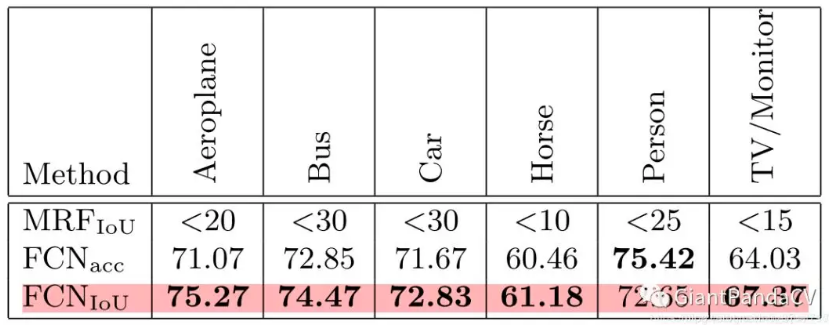## 6. Tversky Loss

Tversky 系数的定义，它是 Dice系数和 Jaccard系数（就是IOU系数，即$\frac{A \bigcap B}{A \bigcup B}$）的广义系数, 公式为：

$$T(A, B) = \frac{A \bigcap B}{A \bigcap B + \alpha |A - B| + \beta |B-A|}$$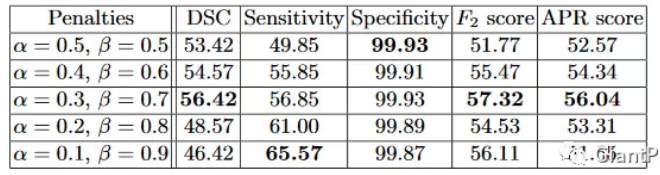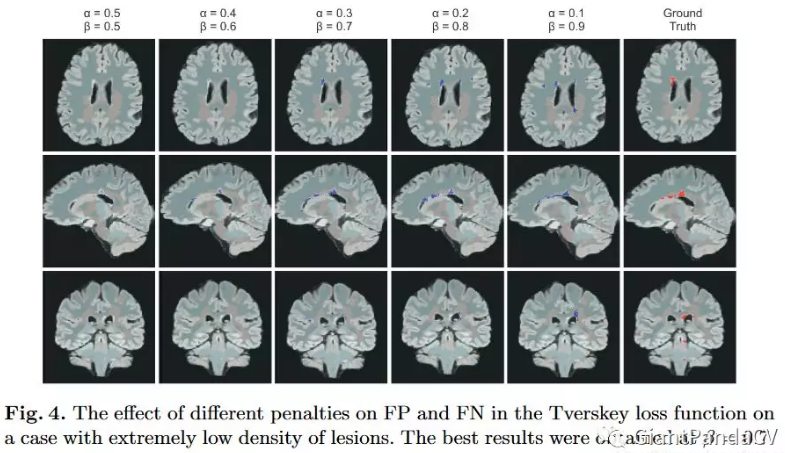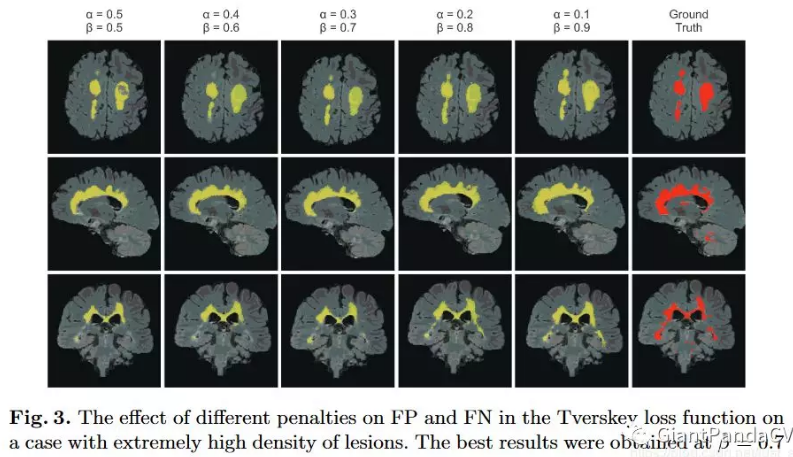Keras 实现如：

def tversky(y_true, y_pred):
y_true_pos = K.flatten(y_true)
y_pred_pos = K.flatten(y_pred)
true_pos = K.sum(y_true_pos * y_pred_pos)
false_neg = K.sum(y_true_pos * (1-y_pred_pos))
false_pos = K.sum((1-y_true_pos)*y_pred_pos)
alpha = 0.7
return (true_pos + smooth)/(true_pos + alpha*false_neg + (1-alpha)*false_pos + smooth)

def tversky_loss(y_true, y_pred):
return1 - tversky(y_true,y_pred)

## 7. Generalized Dice loss

Generalized Dice loss 代码实现：https://github.com/keras-team/keras/issues/9395

GDL Loss在类别数为2时公式如下：

$$GDL = 1-2\frac{\sum_{l=1}^2 w_l \sum_n r_{ln} p_{ln}}{\sum_{l=1}^2 w_l \sum_n r_{ln} + p_{ln}}$$

Keras 实现：

def generalized_dice_coeff(y_true, y_pred):
Ncl = y_pred.shape[-1]
w = K.zeros(shape=(Ncl,))
w = K.sum(y_true, axis=(0,1,2))
w = 1/(w**2+0.000001)
# Compute gen dice coef:
numerator = y_true*y_pred
numerator = w*K.sum(numerator,(0,1,2,3))
numerator = K.sum(numerator)
denominator = y_true+y_pred
denominator = w*K.sum(denominator,(0,1,2,3))
denominator = K.sum(denominator)
gen_dice_coef = 2*numerator/denominator
return gen_dice_coef

def generalized_dice_loss(y_true, y_pred):
return1 - generalized_dice_coeff(y_true, y_pred)

## 9. Focal Loss + Dice Loss

https://arxiv.org/pdf/1808.05238.pdf

$$TP_p(c) = \sum_{n=1}^N p_n(c) g_n(c)$$

$$FN_p(c) = \sum_{n=1}^N (1 - p_n(c)) g_n(c)$$

$$FP_p(c) = \sum_{n=1}^N p_n(c)(1 - g_n(c))$$

$$\mathcal{L} = \mathcal{L}_{Dice} + \lambda \mathcal{L}_{Focal}$$

$$\mathcal{L} = C - \sum_{c=0}^{C-1} \frac{TP_p(c)}{TP_p(c) + \alpha FN_p(c) + \beta FP_p(c)} - \lambda \frac{1}{N} \sum_{c=0}^{C-1} \sum_{n=1}^{N}g_n(c)(1 - p_n(c))^2log(p_n(c))$$

## 10. Exponential Logarithmic loss

MICCAI 2018 论文 3D Segmentation with Exponential LogarithmicLoss for Highly Unbalanced Object Sizes 所提出来的. 其结合了 Focal Loss以及Dice loss:

$$L_{Exp} =w_{Dice}L_{Dice} + w_{Cross}L_{Cross}$$

$$L_{Dice} = \mathbf{E}[(-ln(Dice_i))^{\gamma Dice}]$$

$$Dice_i = \frac{2(\sum_{\mathbf{x}}\sigma_{il}(\mathbf{x})p_i(\mathbf{x})) + \epsilon}{(\sum_{\mathbf{x}}\sigma_{il}(\mathbf{x}) + p_i(\mathbf{x})) + \epsilon}$$

$$L_{Cross} = \mathbf{E}[w_l(-ln(p_l(\mathbf{x})))^{\gamma Cross}]$$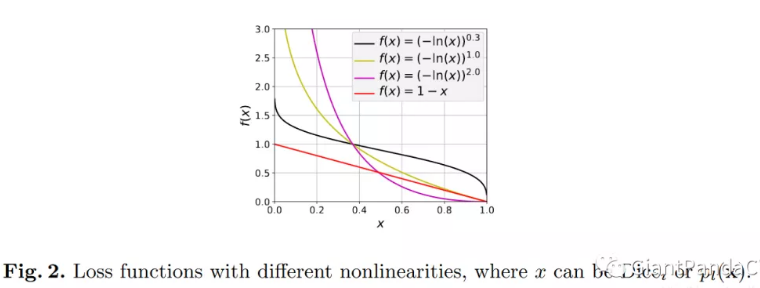## 11. Lovasz-Softmax Loss

Github - LovaszSoftmax 给出了Pytorch 和 Tensorflow 的实现.

HomePage - LovaszSoftmax

Lovasz-Softmax Loss是对 Jaccard(IOU) Loss进行 Lovaze扩展，表现更好.

## 12. 补充 - Softmax 梯度计算

Softmax 函数，如：

$$S_i = \frac{e^{z_i}}{\sum_k e^{z_k}}$$

$$z_i = \sum_{ij} x_{ij} + b$$

$$a_i = \frac{e^{z_i}}{\sum_k e^{z_k}}$$

$$C = -\sum_i y_i ln a_i$$

CS231N 给出一份代码实现：

#coding=utf-8
import numpy as np

def softmax_loss_native(W, X, y, reg):
'''
Softmax_loss的暴力实现，利用for循环
输入的维度是D，有C个分类类别，在有N个例子的batch上进行操作
输入:
- W: 一个numpy array，形状是(D, C)，代表权重
- X: 一个形状为(N, D)为numpy array，代表输入数据
- y: 一个形状为(N,)的numpy array，代表类别标签
- reg: (float)正则化参数
f返回:
- 一个浮点数代表Loss
- 和W形状一样的梯度
'''
loss = 0.0
dW = np.zeros_like(W) #dW代表W反向传播的梯度
num_classes = W.shape
num_train = X.shape
for i in xrange(num_train):
scores = X[i].dot(W)
shift_scores = scores - max(scores) #防止数值不稳定
loss_i = -shift_scores[y[i]] + np.log(sum(np.exp(shift_scores)))
loss += loss_i
for j in xrange(num_classes):
softmax_output = np.exp(shift_scores[j]) / sum(np.exp(shift_scores))
if j == y[i]:
dW[:, j] += (-1 + softmax_output) * X[i]
else:
dW[:, j] += softmax_output * X[i]
loss /= num_train
loss += 0.5 * reg * np.sum(W * W)
dW = dW / num_train + reg * W
return loss, dW

def softmax_loss_vectorized(W, X, y, reg):
loss = 0.0
dW = np.zeros_like(W)
num_class = W.shape
num_train = X.shape
scores = X.dot(W)
shift_scores = scores - np.max(scores, axis=1).reshape(-1, 1)
softmax_output  = np.exp(shift_scores) / np.sum(np.exp(shift_scores), axis=1).reshape(-1, 1)
loss = -np.sum(np.log(softmax_output[range(num_train), list(y)]))
loss /= num_train
loss += 0.5 * reg * np.sum(W * W)
dS = softmax_output.copy()
dS[range(num_train), list(y)] += -1
dW = (x.T).dot(dS)
dW = dW/num_train + reg*W
return loss, dW
Last modification：February 14th, 2020 at 11:11 am